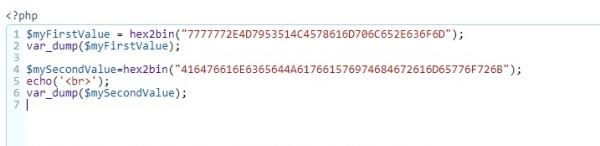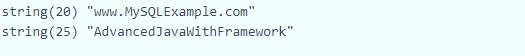# What is the PHP equivalent of MySQL's UNHEX()?

You can use hex2bin() function since it is the PHP equivalent of MySQL's UNHEX().

The syntax is as follows −

$anyVariableName = hex2bin("yourHexadecimalValue"); To understand the above syntax, let us implement the above syntax in PHP. The PHP code is as follows − $myFirstValue = hex2bin("7777772E4D7953514C4578616D706C652E636F6D");
var_dump($myFirstValue);$mySecondValue=hex2bin("416476616E6365644A617661576974684672616D65776F726B");
echo('<br>');
var_dump(\$mySecondValue);

The snapshot of PHP code is as follows −Here is the snapshot of The output −Here is the MySQL UNHEX() −

Case 1 − The query is as follows −

mysql> SELECT UNHEX("7777772E4D7953514C4578616D706C652E636F6D");

The following is The output −

+---------------------------------------------------+
| UNHEX("7777772E4D7953514C4578616D706C652E636F6D") |
+---------------------------------------------------+
| www.MySQLExample.com                              |
+---------------------------------------------------+
1 row in set (0.00 sec)

Case 2 − The query is as follows −

mysql> SELECT UNHEX("416476616E6365644A617661576974684672616D65776F726B");

Here is the output −

+-------------------------------------------------------------+
| UNHEX("416476616E6365644A617661576974684672616D65776F726B") |
+-------------------------------------------------------------+
1 row in set (0.00 sec)